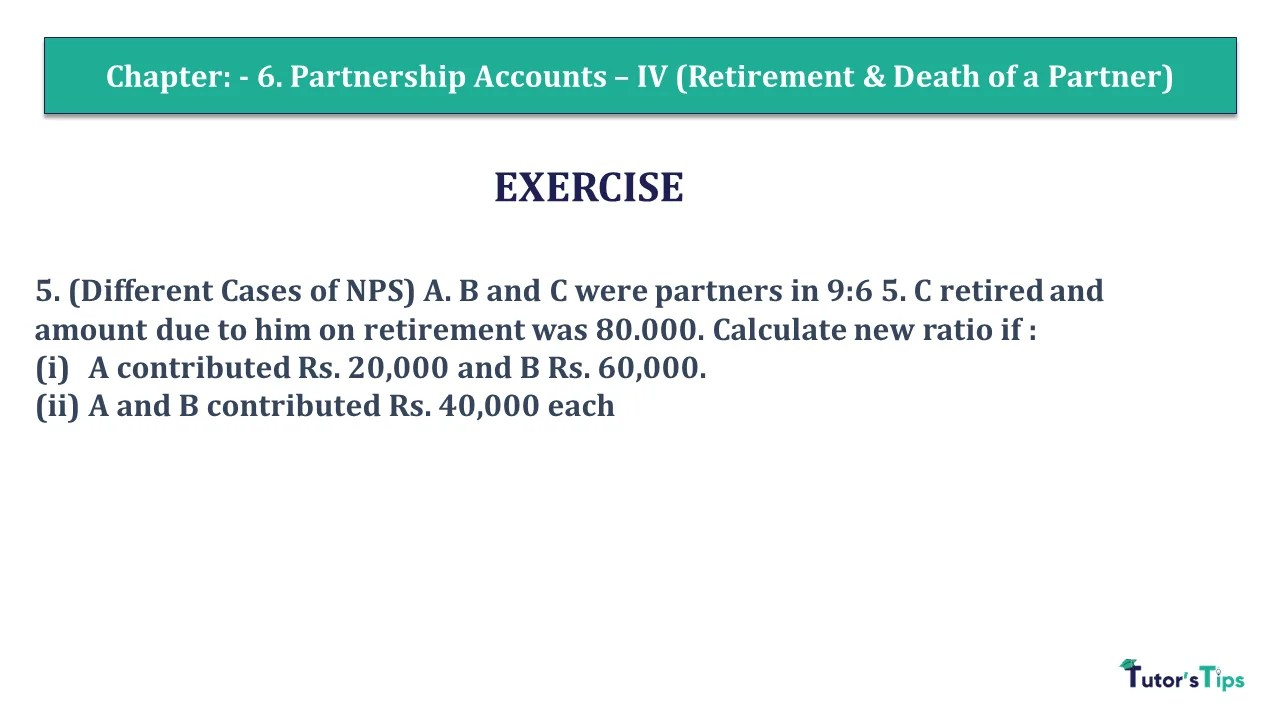# Question 05 Chapter 6 of +2 Part-1 – USHA Publication 12 Class Part – 1Q-5 - CH-6 - Usha +2 Book 2018 - Solution

Question 05 Chapter 6 of +2-Part-1

5. (Different Cases of NPS) A. B and C were partners in 9:6 5. C retired and amount due to him on retirement was 80.000. Calculate new ratio if :
(i) A contributed Rs. 20,000 and B Rs. 60,000.
(ii) A and B contributed Rs. 40,000 each

## The solution of Question 05 Chapter 6 of +2 Part-1: –

Calculation of new Profit Sharing ratio

Contribution made by A & B is Rs. 20,000 & Rs. 60,000 respectively .

Gaining ratio = 20,000 : 60,0000 = 1 : 3

 A’s gain = 1 x 5 4 20 = 1 16
 & B’s gain = 3 x 5 4 20 = 3 16
 Thus A’s New Share = 9 + 1 20 16 = 41 80
 And B’s New Share = 6 x 3 20 16 = 39 80
 So, New Profit sharing Ratio = 41 : 39 80 80 = 41 : 39

(ii) Contribution made by A & B is Rs. 40,000 (each ).

Gaining ratio = 1 : 1

 A’s gain = 1 x 5 2 20 = 1 8
 & B’s gain = 1 x 5 2 20 = 1 8
 Thus A’s New Share = 1 + 9 8 20 = 23 40
 And B’s New Share = 1 x 6 8 20 = 17 40
 So, New Profit sharing Ratio = 23 : 17 40 40 = 23 : 17

Comment if you have any questions.

Also, Check out the solved question of previous Chapters: –